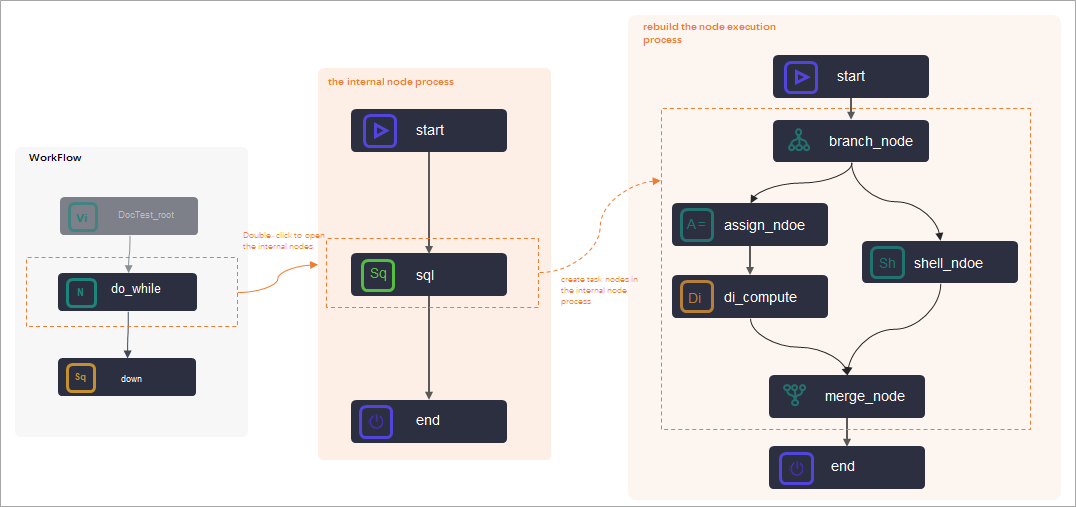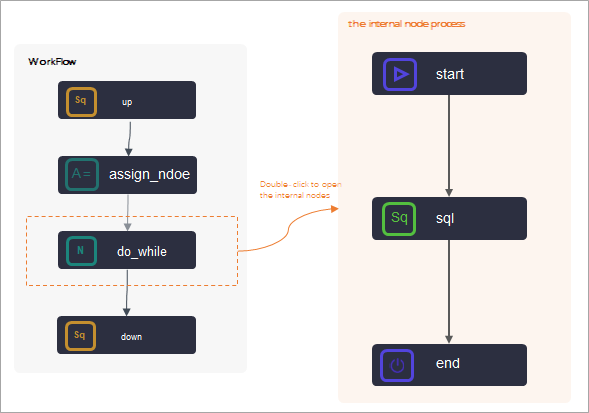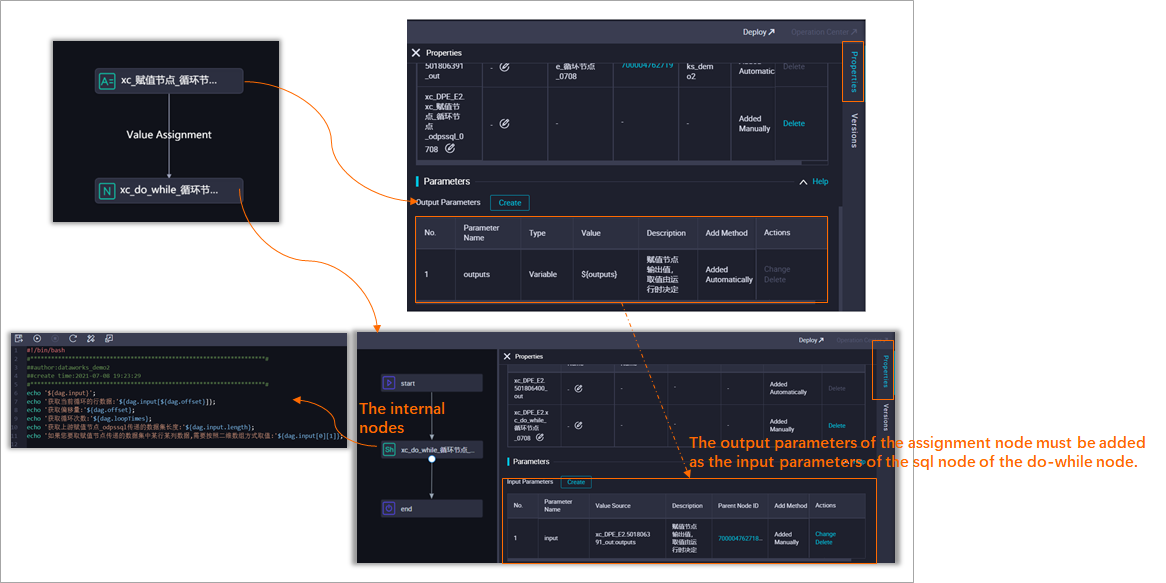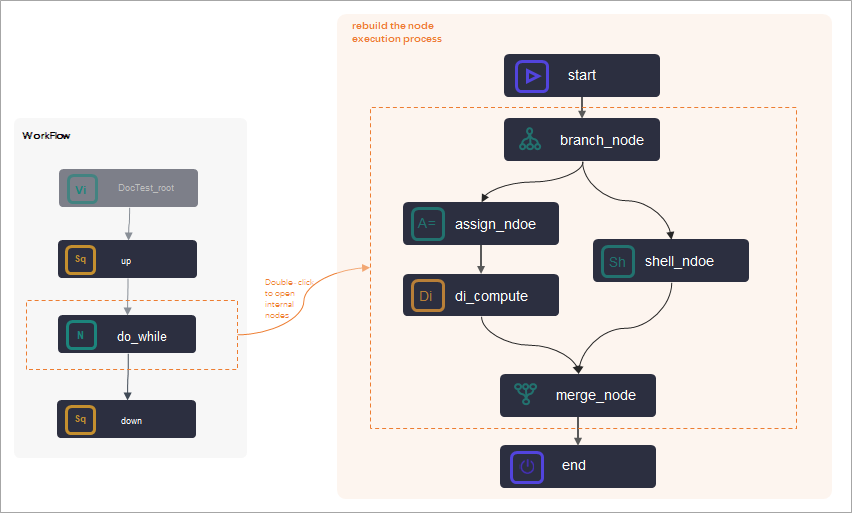DataWorks provides do-while nodes. You can rearrange the workflow inside a do-while node, write the logic to be executed in a loop in the node, and then configure an end node to determine whether to exit the loop. You can also use a do-while node together with an assignment node to loop through the result set that is passed by the assignment node. This topic describes the composition and application logic of do-while nodes.

## Node composition

A do-while node in DataWorks is a special node that contains internal nodes. When you create a do-while node, the following three internal nodes are automatically created: the start node (loop start node), the sql node (loop task node), and the end node (loop end node). The internal nodes are organized into an internal node process to perform the task in a loop.The preceding figure shows the following information:
• start node

The start node in the internal nodes does not carry specific task code.

• sql node
By default, DataWorks creates an internal SQL task node. You can delete the default sql node and customize internal loop task nodes.
• If your loop task is an SQL task, you can double-click the default sql node to go to the node configuration tab to develop loop task code.
• If your loop task is complex, you can create task nodes in the internal node process and rebuild the node execution process based on actual situations.
Generally, a do-while node is used together with an assignment node, a branch node, and a merge node. For more information about typical scenarios, see Typical scenario: Use a do-while node together with an assignment node.
Note When you customize a do-while node, you can delete the dependencies between the internal nodes and rearrange the internal workflow of the do-while node. However, you must use the start node and the end node as the start and end nodes of the internal workflow of the do-while node.
• end node
• The end node is used to determine the number of times the loop is run for the do-while node. The end node is essentially an assignment node. The end node returns `true` or `false`. true indicates to run the loop again, and false indicates to exit the loop.
• You can use MaxCompute SQL, Shell, or Python 2 to develop the code of the end node. The do-while node provides convenient built-in variables for you to develop the end code. For more information about built-in variables, see Built-in variables and Examples of variable values. For more information about sample codes developed in different languages, see Example 1: Sample code of the end node.

## Limits and usage nodes

• Support for do-while nodes
• You can use do-while nodes only in DataWorks Standard Edition or a more advanced edition.
• A do-while node supports a maximum of 128 times the loop is run. If the number of times the loop is run determined by the end node exceeds 128, an error is returned.
• Internal nodes
• When you customize a do-while node, you can delete the dependencies between the internal nodes and rearrange the internal workflow of the do-while node. However, you must use the start node and the end node as the start and end nodes of the internal workflow of the do-while node.
• When the internal nodes of a do-while node use a branch node to perform logical judgments or traverse results, a merge node also needs to be used.
• Test and running
• If the workspace is in standard mode, you cannot directly test and run a do-while node in DataStudio.

To test the do-while node and view the result, you must commit the do-while node to Operation Center and run the do-while node in Operation Center. If you use the value passed by an assignment node in the do-while node, run both the assignment node and do-while node during the test in Operation Center.

• When you view the operational logs of a do-while node in Operation Center, right-click the do-while node and select View Internal Nodes to view the operational logs of the internal nodes.

## Typical scenario: Use a do-while node together with an assignment node

A do-while node is often used together with an assignment node, as shown in the following figure.When you use a do-while node together with an assignment node:
• You must use the output of the assignment node as the input of the do-while node, and configure the dependencies of the do-while node on the assignment node. For more information about usage notes, see Example 2: Use a do-while node together with an assignment node.
• You can use some built-in variables to obtain the current number of times the loop is run and the values of the assignment parameters. For more information, see Built-in variables.

## Built-in variables

A do-while node in DataWorks uses internal nodes to run a task in a loop. Each time the task is run in a loop, you can use some built-in variables to obtain the current number of times the loop is run and the offset.
Built-in variable Description Value
`\${dag.loopTimes}` The current number of times the loop is run. 1 when the loop is run for the first time, 2 when the loop is run for the second time, 3 when the loop is run for the third time, ..., and n when the loop is run for the nth time.
`\${dag.offset}` The offset. 0 when the loop is run for the first time, 1 when the loop is run for the second time, 2 when the loop is run for the third time, ..., and n-1 when the loop is run for the nth time.
If you use a do-while node together with an assignment node, you can also obtain the values of the assignment parameters and loop variables in the following way:
Note In the following variable examples, `input` specifies the name of the input parameter defined in the do-while node. You must replace input with the actual name of the input parameter.
Built-in variable Description
`\${dag.input}` The dataset passed by the parent assignment node.
`\${dag.input[\${dag.offset}]}` The data row obtained by the do-while node in the current loop.
`\${dag.input.length}` The length of the dataset obtained inside the do-while node.

## Examples of variable values

• Example 1
The parent assignment node is a Shell node, and the last output is `2021-03-28,2021-03-29,2021-03-30,2021-03-31,2021-04-01`. The following table describes the values of the variables in Example 1.
Built-in variable Value when the loop is run for the first time Value when the loop is run for the second time
`\${dag.input}` `2021-03-28,2021-03-29,2021-03-30,2021-03-31,2021-04-01`
`\${dag.input[\${dag.offset}]}` `2021-03-28` `2021-03-29`
`\${dag.input.length}` 5
`\${dag.loopTimes}` 1 2
`\${dag.offset}` 0 1
• Example 2
The parent assignment node is an ODPS SQL node, and the last SELECT statement queries the following two pieces of data:
``````+----------------------------------------------+
| uid            | region | age_range | zodiac |
+----------------------------------------------+
| 0016359810821  | Hubei Province | 30–40 years old   | Cancer (constellation) |
| 0016359814159  | Unknown   | 30–40 years old   | Cancer (constellation) |
+----------------------------------------------+``````
The following table describes the values of the variables in Example 2.
Built-in variable Value when the loop is run for the first time Value when the loop is run for the second time
`\${dag.input}`
``````+----------------------------------------------+
| uid            | region | age_range | zodiac |
+----------------------------------------------+
| 0016359810821  | Hubei Province | 30–40 years old   | Cancer (constellation) |
| 0016359814159  | Unknown   | 30–40 years old   | Cancer (constellation) |
+----------------------------------------------+``````
`\${dag.input[\${dag.offset}]}` `0016359810821, Hubei Province, 30–40 years old, Cancer (constellation)` `0016359814159, unknown, 30–40 years old, Cancer (constellation)`
`\${dag.input.length}` 2
Note The number of rows in a two-dimensional array is the length of the dataset. The number of rows in a two-dimensional array in the output of the assignment node is 2.
`\${dag.input`
Note The value in the first row and first column of the two-dimensional array.
`0016359810821`
`\${dag.loopTimes}` 1 2
`\${dag.offset}` 0 1

## Example 1: Sample code of the end node

You can use MaxCompute SQL, Shell, or Python 2 to develop the code of the end node. The following part shows typical sample code in these three different languages.
• MaxCompute SQL:
``````SELECT  CASE
WHEN COUNT(1) > 0 AND \${dag.offset}<= 9
THEN true
ELSE false
END
FROM  xc_dpe_e2.xc_rpt_user_info_d  where dt='20200101';``````

In the preceding sample code of the end node, the number of rows and the offset are compared with fixed values to limit the number of times the loop is run for the do-while node.

• Shell:
``````if [ \${dag.loopTimes} -lt 5 ];
then
echo "True"
else
echo "False"
fi``````

In the preceding code, the number of times the loop is run is compared with 5 to limit the number of times the loop is run for the do-while node. The `\${dag.loopTimes}` variable specifies the number of times the loop is run.

The value of the `\${dag.loopTimes}` variable is 1 when the loop is run for the first time and is incremented by 1 each time, for example, 2 for the second time, and 5 for the fifth time. At this point, the output of the end node is false, and the do-while node exits the loop.

• Python 2:
``````if \${dag.loopTimes}<\${dag.input.length};
print True;
else
print False;
# Run the loop again if the end node returns True.
# Exit the loop if the end node returns False. ``````

In the preceding code, the number of times the loop is run is compared with the number of rows in the dataset passed by the assignment node to limit the number of times the loop is run for the do-while node. The `\${dag.loopTimes}` variable specifies the number of times the loop is run.

## Example 2: Use a do-while node together with an assignment node

The following table describes a typical scenario and usage notes for using a do-while node together with an assignment node.Scenario Usage note Configuration example
When a `do-while` node is used to perform a loop task, each time the loop is run, the internal nodes need to obtain and use the output parameters of the parent node (`up node`). In this case, you can use an assignment node (`assign_node`).
• Dependencies

The do-while node depends on the parent assignment node (assign_node).

Note The do-while node rather than the `sql` node of the do-while node depends on the assignment node, as shown in the preceding figure.
• Context parameters
• The assignment node must use the output parameters as the output parameters of the assignment node (`assign_node`).
• The output parameters of the assignment node must be added as the input parameters of the `sql` node of the do-while node.
Note You must set context relationships for the internal loop task node rather than the do-while node.## Example 3: Use a do-while node together with a branch node and a merge node

The following table describes a typical scenario and usage notes for using a do-while node together with a branch node and a merge node.Scenario Usage note
The do-while node needs to perform logical judgment or result traversal. In this case, you can customize the loop task node in the internal node of the do-while node and use a branch node (`branch_node`) and a merge node (`merge_node`). In the do-while node, the branch node (`branch_node`) and the merge node (`merge_node`) need to be used at the same time.